# SSC CGL Algebra Questions: Solve Important Questions Here | Part 1

The SSC is going to conduct SSC CGL  2019-20 Tier 1 Examination from 2 March 2020 to 11 March 2020 and candidates appearing would be totally embraced with their preparations. SSC CGL is one of the toughest examinations conducted by SSC every year and when we talk about the toughness of the section, the Quantitative aptitude section is often considered to be on top. Questions from Algebra are commonly asked in SSC CGL examination every year and this topic is considered to be one of the important topics. Keeping in mind the importance and toughness of this topic, we have brought you some Important SSC CGL Algebra Questions which are probable to appear in the upcoming SSC CGL examination. Let’s start with the blog, SSC CGL Algebra Questions Part 1.

Attempt More Important Algebra Questions Here

## SSC CGL Algebra Questions: Solve Practice Questions Here

Q1) If a = 1.21, b = 2.12 and c = –3.33 then, the value of a³+ b³+ c³– 3abc is?

A. O
B. 1
C. 2
D. 3

Q2) If a/b = b/4 = c/7, then (a+b+c) / c is equal to?

A. O
B. 1
C. 2
D. 3

Check where you stand with your preparation by taking a mock test

Q3) If a = 0.25, b = – 0.05, c = 0.5, then the value of ( a²-b²-c²-2bc ) / (a²-b²-2ab-c²) is?

A.7/8
B. 14/17
C. 1
D. 25/16

Q4) If, (√7-2) / (√7+2) – a√7+b, then the value of a is?

A. 11/3
B. -4/3
C. 4/3
D. -(4√7)/3

Check where you stand with your preparation by taking a mock test

Q5) If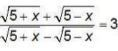, then what is the value of x?

A. 5/2
B. 25/3
C. 4
D. 3

Q6) If (x + y + z) = 12, xy + yz + zx = 44 and xyz = 48,then what is the value of x³+y³+z³= ?

A.104
B. 144
C. 196
D. 288

Try Best Algebra Questions here Important For SSC CGL Examination

Q7) If x(2x + 3) = 90 and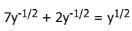(x and y are positive numbers), then what is the value of x²+y²?

A. 45
B. 109
C. 117
D.  126

Q8) If x²+y²+z² = xy + yz + zx, then what is the value of (7x + 3y – 5z)/5x?

A. 0
B. 1
C. 5
D. 33/5

Try More Important Algebra Questions Here

Q9)  If a + b + c = 11 and ab + bc + ca = 17, then what is the value of a³+b³+c³-3abc?

A. 121
B. 168
C. 300
D. 770

Q10) If (x/5) + (5/x) = – 2, then what is the value of x³ ?

A. -125
B. 5
C. 1/125
D.  625

Attempt More SSC CGL Quantitative Aptitude Questions Here

Q11)  If x + (1/x)  = 2,then what is the value of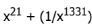?

A. 0
B. 1
C. 2
D.  4

Q12) If x+y+z = 13 and x²+y²+z²=69, then xy+z(x+y)=?

A. 50
B. 60
C. 100
D. 80

Attempt More SSC CGL Quantitative Aptitude Questions Here

Q13) If x + (a/x) = 1, then the value of (x²+x+a) / (x³-x²) = ?

A. -2/a
B. 2/a
C. 1/a
D. -1/a

Q14) If (m-a²) / (b²+ c²) + (m-b² )/ (c²+a²) + (m-c²)/ (a²+b²) = 3, the value of m?

A. ab+bc+ca-abc
B. a²+b²+c²
C.(a+b+c)²
D.a²+b²+cab-bc-ca

Attempt More SSC CGL Quantitative Aptitude Questions Here

Q15)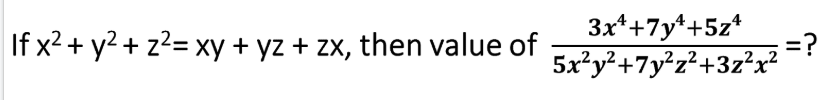A. 0
B. 1
C. 2
D. 3

Q16)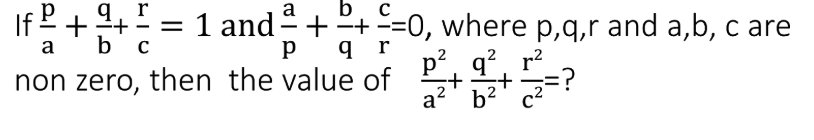A. 1
B. 2
C. 3
D. 0

Try More Important Algebra Questions Here

Q17) If a/b = b/c = c/d, then the value of (b-c)²+(c-a)²+(d-b)²=?

A. a+d
B. a-d
C. (a+d)²
D. (a-d)²

Q18)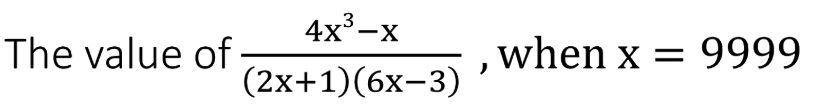A. 222
B. 111
C. 444
D. 333

Try More Important Algebra Questions Here

Q19) If (1/x) + (1/y) + (1/z) = 0 and x + y + z = 9, then what is the value of x³+y³+z³-3xyz ?

A. 81
B. 361
C. 729
D. 6561

Q20) If x + 1/(x+2) = 6, then the value of (x+2)³ + 1/(x+2)³?

A. 512
B. 488
C. 486
D. 500